Home Practice
For learners and parents For teachers and schools
Textbooks
Full catalogue
Pricing SupportLog in

We think you are located in United States. Is this correct?

# Test yourself now

High marks in science are the key to your success and future plans. Test yourself and learn more on Siyavula Practice.

## 26.2 Probability terminology

We will use three examples of uncertain processes (called experiments) to explain the meanings of the different words that we use in probability: tossing a coin, rolling dice, and selecting a card from a pack of cards.

experiment
a test or activity that can be repeated many times, and that has a certain number of possible outcomes

### Examples of experiments

1. Tossing a fair coin

There are two possible outcomes: the coin will either land with heads ($$H$$) or with tails ($$T$$) facing upwards. An example outcome of tossing a coin is that it lands with heads facing up.

fair
outcome
a single result of an experiment
2. Rolling a fair dice

A dice has six sides numbered from $$1$$ to $$6$$, so there are six possible outcomes. An example outcome of rolling a dice is getting a $$5$$.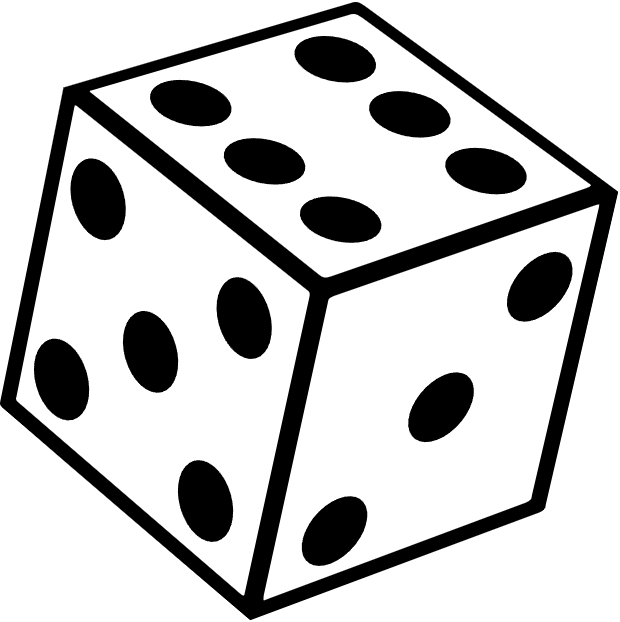3. Drawing a random card from a pack of cards

There are 52 cards in a pack. An example outcome of drawing a random card from the pack is getting the queen of hearts card.

random
when something happens without being made to happen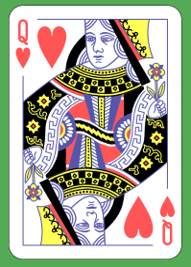Dmitry Fomin, CC0, via Wikimedia Commons

The sample space of an experiment is the set of all possible outcomes of that experiment. The symbol $$S$$ is used for the sample space, and the size of the sample space (the total number of possible outcomes) is written as $$n(S)$$.

sample space
the set of all possible outcomes of that experiment
size of the sample space
the total number of possible outcomes

Even though we are usually interested in the outcome of an experiment, we also need to know what the other outcomes could have been.

### Sample spaces of experiments

1. A coin can land heads up or tails up, so the sample space is the set $$S = {H; T}$$.

The size of the sample space of the coin toss is $$n(S) = 2$$.

2. A dice can land on a number from $$1$$ to $$6$$, so the sample space is $$S = {1; 2; 3; 4; 5; 6}$$.

The number of possible outcomes is $$n(S) = 6$$.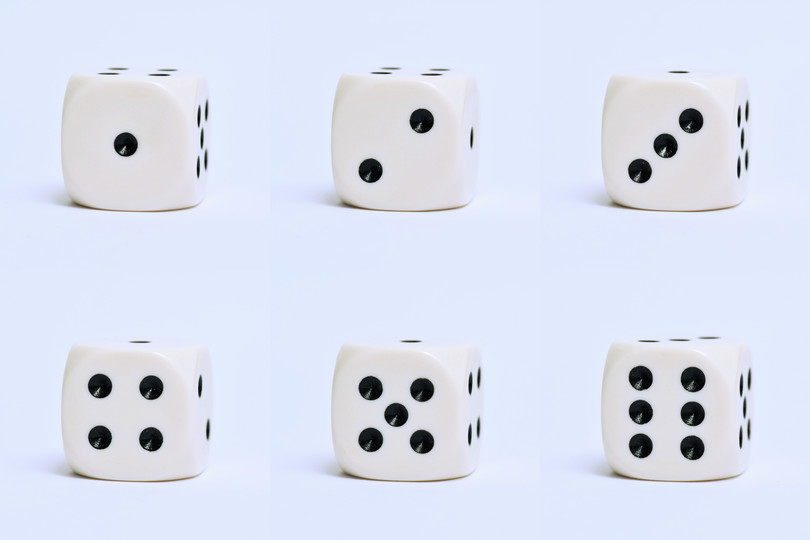3. There are $$52$$ cards in a pack of cards. A pack of playing cards is made up of four different suits: spades ($$\spadesuit$$), diamonds ($$\diamondsuit$$), clubs ($$\clubsuit$$) and hearts ($$\heartsuit$$). Clubs and spades are usually black and hearts and diamonds are usually red.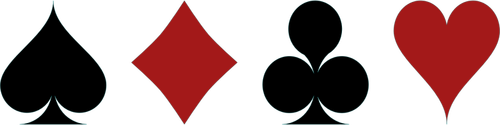Each suit has thirteen cards: ace ($$A$$), $$2$$, $$3$$, $$4$$, $$5$$, $$6$$, $$7$$, $$8$$, $$9$$, $$10$$, jack ($$J$$), queen ($$Q$$) and king ($$K$$).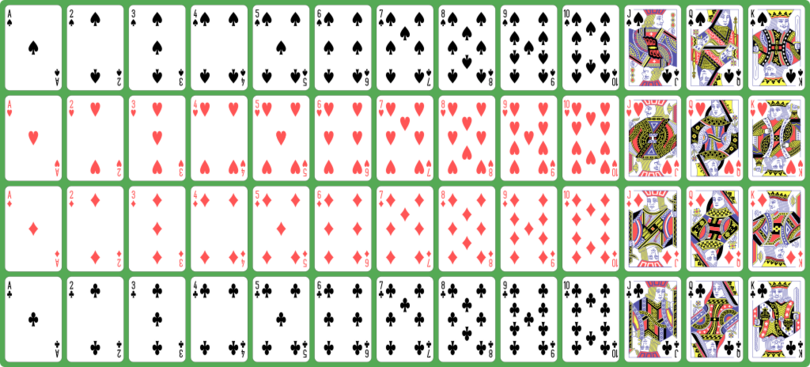Dmitry Fomin, CC0, via Wikimedia Commons

When drawing a single card at random from a pack of cards, the sample space is:

\begin{align} S =& \\ &{2\spadesuit; 3\spadesuit; 4\spadesuit; 5\spadesuit; 6\spadesuit; 7\spadesuit; 8\spadesuit; 9\spadesuit; 10\spadesuit; J\spadesuit; Q\spadesuit; K\spadesuit; A\spadesuit;} \\ &{2\diamondsuit; 3\diamondsuit; 4\diamondsuit; 5\diamondsuit; 6\diamondsuit; 7\diamondsuit; 8\diamondsuit; 9\diamondsuit; 10\diamondsuit; J\diamondsuit; Q\diamondsuit; K\diamondsuit; A\diamondsuit;} \\ &{2\clubsuit; 3\clubsuit; 4\clubsuit; 5\clubsuit; 6\clubsuit; 7\clubsuit; 8\clubsuit; 9\clubsuit; 10\clubsuit; J\clubsuit; Q\clubsuit; K\clubsuit; A\clubsuit;} \\ &{2\heartsuit; 3\heartsuit; 4\heartsuit; 5\heartsuit; 6\heartsuit; 7\heartsuit; 8\heartsuit; 9\heartsuit; 10\heartsuit; J\heartsuit; Q\heartsuit; K\heartsuit; A\heartsuit} \end{align}

The number of possible outcomes is $$n(S) = 52$$.

To draw a card from the pack means to pull out one random card from the pack.

An event ($$E$$) is a specific set of outcomes of an experiment that you are interested in. The number of outcomes in an event is written as $$n(E)$$.

event
a specific set of outcomes of an experiment that you are interested in

For example, you toss a coin and want it to land heads up (because if it lands tails up, you have to wash the dishes). Here, the event contains a single outcome: $$E={H}$$. The size of the event set is $$n(E) = 1$$.

Let us say that we roll a dice and we are interested in the face of the dice being less than $$4$$. In this case the event set is $$E = {1; 2; 3}$$ and $$n(E) = 3$$.

We draw a card from a pack and we would like to pull out a heart card. Here the event set contains thirteen possible outcomes: $$E = {2\heartsuit; 3\heartsuit; 4\heartsuit; 5\heartsuit; 6\heartsuit; 7\heartsuit; 8\heartsuit; 9\heartsuit; 10\heartsuit; J\heartsuit; Q\heartsuit; K\heartsuit; A\heartsuit}$$.
The number of outcomes in the event $$n(E) = 13$$.